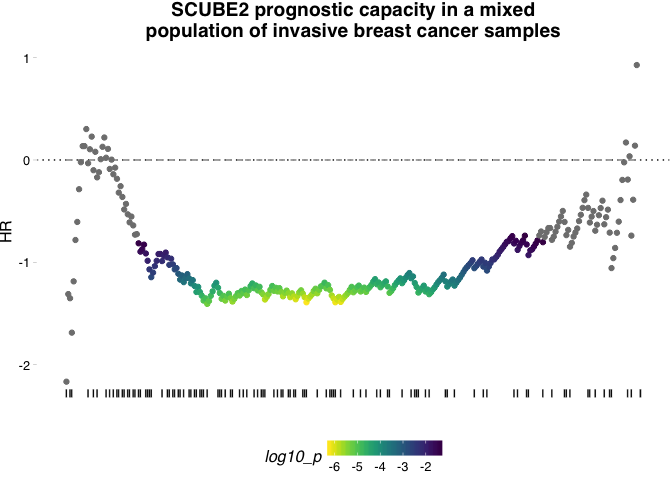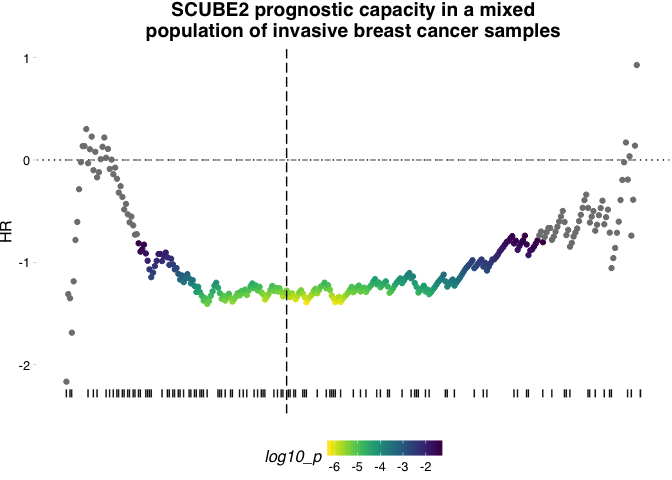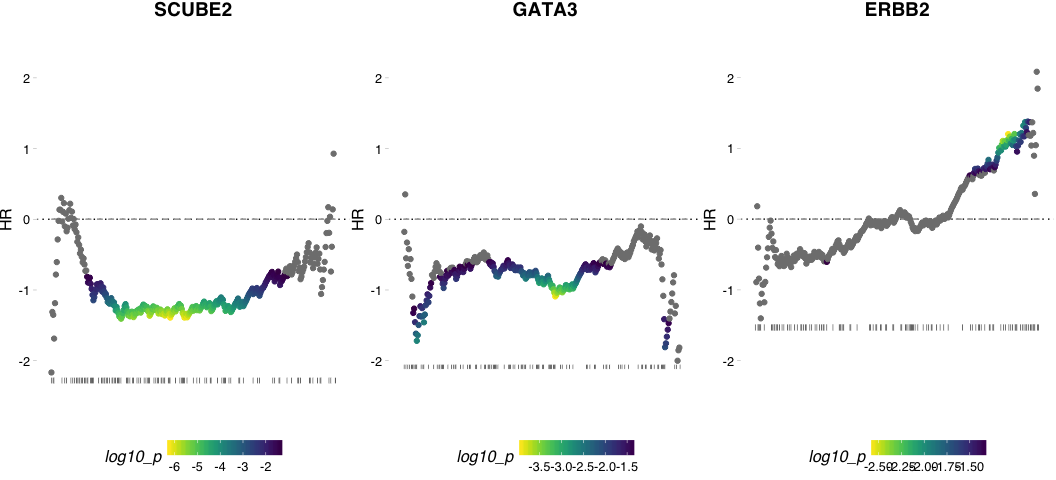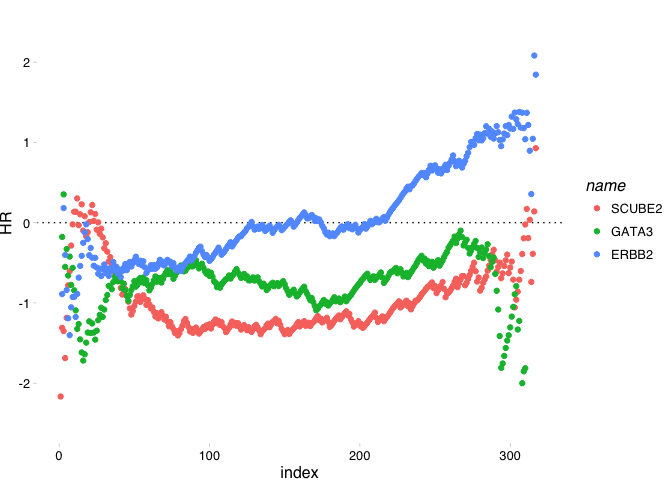# Basic Usage

Dominic Pearce, The Institute of Genetics and Molecular Medicine, The University of Edinburgh
2018-04-25

#### Quick example using NKI breast cancer data

note: this data is pre-subsetted to only include patients with complete distant metastasis information (e.dmfs & t.dmfs)

``````library(survivALL)
library(Biobase)
library(ggplot2)

data(nki_subset)``````

## Example : Plotting

We use a continuous measure, here a vector of gene expression, to re-order our survival data and then compute hazard ratios and pvalues for all points of separation

``````xpr_vec <- exprs(nki_subset)["NM_020974", ] #expression vector for SCUBE2 (anti-correlated with proliferation)

plotALL(
measure = xpr_vec, #expression data
srv = pData(nki_subset), #survival information
time = "t.dmfs", #time-to-outcome
event = "e.dmfs", #outcome type
bs_dfr = c(), #thresholding data would go here
measure_name = "SCUBE2", #our gene's name
title = "SCUBE2 prognostic capacity in a mixed\npopulation of invasive breast cancer samples", #plot title
)``````Note that we can add additional elements using standard `ggplot2` syntax. Here we add a horizontal indicator of the most significant point of separation

``````a_random_x_axis_value <- 123

plotALL(measure = xpr_vec,
srv = pData(nki_subset),
time = "t.dmfs",
event = "e.dmfs",
bs_dfr = c(),
measure_name = "SCUBE2",
title = "SCUBE2 prognostic capacity in a mixed\npopulation of invasive breast cancer samples") +
geom_vline(xintercept = a_random_x_axis_value, linetype = 5)``````### Plotting multiple genes simultaneously

We first organise our measure data, our expression vectors for three genes of interest SCUBE2, FOS and ERBB2 before applying each in a loop, specifying a common and sensible y-axis range using `ggplot2` conventions. (To choose the limits we produce the plots first, select a rational range by eye and then recompute with the newly specified limits). We then combine the figures using the `cowplot::plot_grid()` function.

``````geneset <- data.frame(refseq_id = c("NM_020974", "NM_002051", "NM_004448"), hgnc_id = c("SCUBE2", "GATA3", "ERBB2"), stringsAsFactors = FALSE)

xpr_lst <- lapply(geneset\$refseq_id, function(id){
exprs(nki_subset)[id,]
})
names(xpr_lst) <- geneset\$hgnc_id

plot_lst <- lapply(geneset\$hgnc_id, function(id){
plotALL(
measure = xpr_lst[[id]], #expression data
srv = pData(nki_subset), #survival information
time = "t.dmfs", #time-to-outcome
event = "e.dmfs", #outcome type
bs_dfr = c(), #thresholding data
measure_name = id, #our gene's name
title = id #plot title
) +
ylim(-2.5, 2.5)
})``````
``cowplot::plot_grid(plotlist = plot_lst, nrow = 1)``## Example : Returning a dataframe

Alternatively, we can return only the computed statistics as a dataframe for further calculations, comparisons and manipulations

``````survivall_out <- survivALL(
measure = xpr_vec, #expression data
srv = pData(nki_subset), #survival information
time = "t.dmfs", #time-to-outcome
event = "e.dmfs", #outcome type
bs_dfr = c(), #thresholding data
measure_name = "SCUBE2" #our gene's name
)``````
``head(survivall_out)``
Table continues below
samples event_time event measure HR p
NKI_369 NKI_369 1190 TRUE -1.316 -2.166 0.2303
NKI_226 NKI_226 972 FALSE -1.308 -1.311 0.4321
NKI_175 NKI_175 2774 TRUE -1.301 -1.351 0.2548
NKI_57 NKI_57 839 TRUE -1.29 -1.687 0.09268
NKI_332 NKI_332 2919 FALSE -1.265 -1.186 0.2149
NKI_24 NKI_24 3227 FALSE -1.253 -0.7819 0.3946
p_adj log10_p bsp bsp_adj index name dsr clsf
NKI_369 0.285 NA NA NA 1 SCUBE2 NA 0
NKI_226 0.4925 NA NA NA 2 SCUBE2 NA 0
NKI_175 0.3116 NA NA NA 3 SCUBE2 NA 0
NKI_57 0.1249 NA NA NA 4 SCUBE2 NA 0
NKI_332 0.2691 NA NA NA 5 SCUBE2 NA 0
NKI_24 0.4563 NA NA NA 6 SCUBE2 NA 0

## Analysing and displaying multiple genes

We can return the results for multiple genes as a single dataframe simply by row-binding the results. Organised in this way we can plot multiple hazard ratio distributions as a single figure

``````survivall_lst <- lapply(geneset\$hgnc_id, function(id){
survivALL(
measure = xpr_lst[[id]], #expression data
srv = pData(nki_subset), #survival information
time = "t.dmfs", #time-to-outcome
event = "e.dmfs", #outcome type
bs_dfr = c(), #thresholding data
measure_name = id #our gene's name
)
})

survivall_dfr <- do.call(rbind, survivall_lst)

ggplot(survivall_dfr, aes(x = index, y = HR, colour = name)) +
geom_hline(yintercept = 0, linetype = 3) +
geom_point() +
ylim(-2.5, 2.5) +
ggthemes::theme_pander()``````